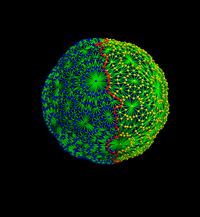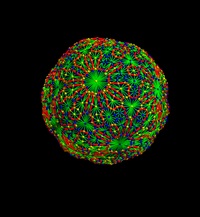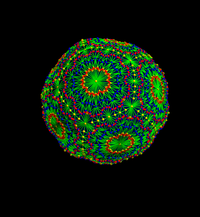# Exhibit: Chladni figures of graphs

Given a finite simple graph, we can look at the eigenfunctions fk of the Laplacian. We are interested in the nodal surfaces {fk=0}. In the case of geometric graphs, graphs for which the unit spheres are spheres, and if the eigenfunction does not take the value 0, our Sard lemma assures that the hyper surface {fk=0} is a d-1 dimensional graph. We visualize these discrete Chladni figures here for 2 spheres. The number of nodal regions is bound by k as a discrete Courant result by Fiedler showed about 50 years ago. We are especially interested in the ground state f2 (the eigenfunction f1 to the eigenvalue 0 is harmonic and so constant). For 2-spheres, the ground state nodal curve is always a circle in the 2-sphere. We conjecture that for 3-sphere graphs, the ground state nodal surface is always a 2 sphere, so that by Jordan Brouwer, the two nodal regions are 3-balls. Even so, the nature of eigenfunctions of the Laplacian has a long tradition starting with Ernst Chladni 1756-1827), we understand little about eigenfunctions of the Laplacian, even the ground state. We are not aware that this question has even be asked in the continuum:

 Is it true that for all Riemannian 3-spheres, the ground state nodal sphere is a 2-sphere? We believe that it is true and that investigating the question in the discrete allows us to get a feel for the problem.

By Courant-Fiedler the ground state f has a nodal surface C = {f=0} which divides the 3 sphere into two 2-balls A,B. Given a function f having a hypersurface C=C(f) dividing the graph into two region A=A(f),B=B(f). Let c(f) = |C(f)|/(|A(f)| |B(f)| denote the Cheeger constant of f, where |C| is the surface area rsp. volume.

 Is it true that for all geometric d-graphs G, the number c(f)2/5 is a lower bound for the ground state energy?

# All Chladni figures of the Icosahedron

Click for larger pictures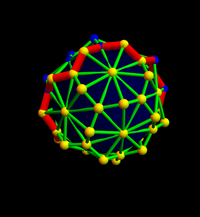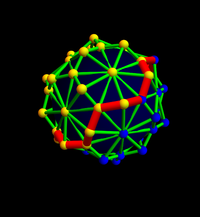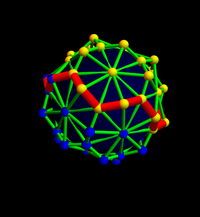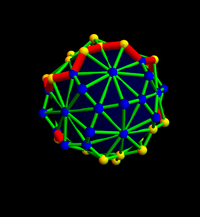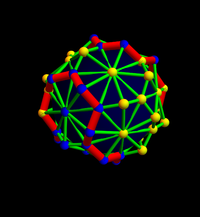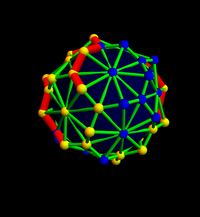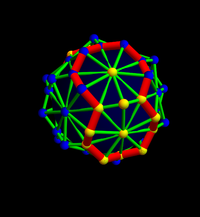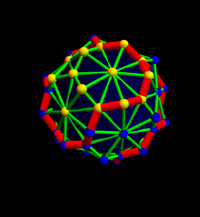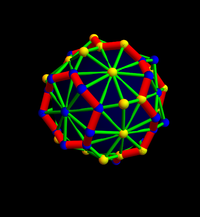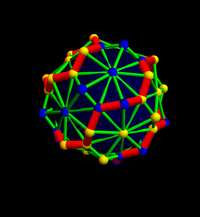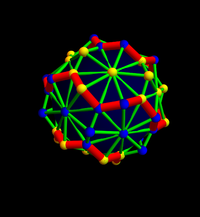# All Chladni figures of a refined Icosahedron

Click for larger pictures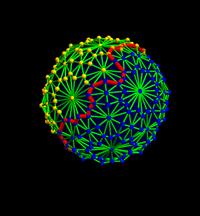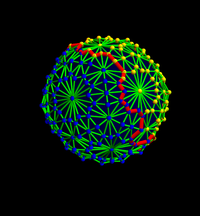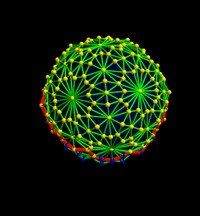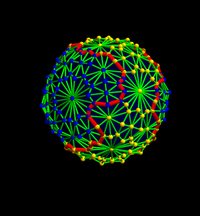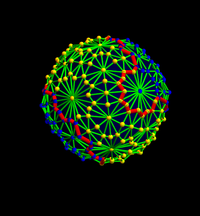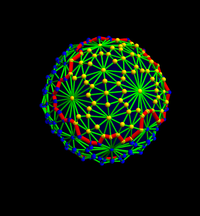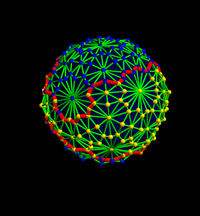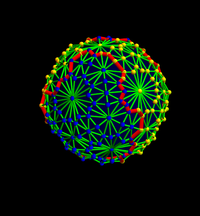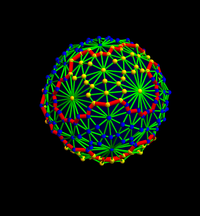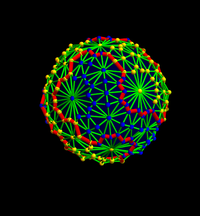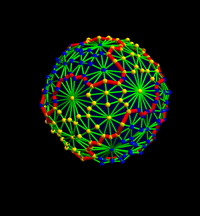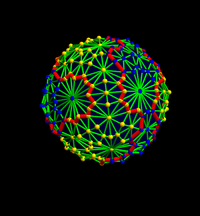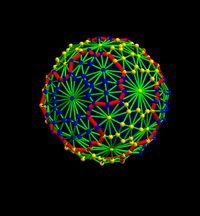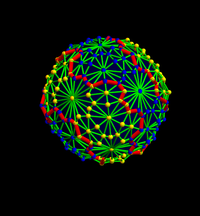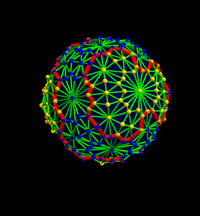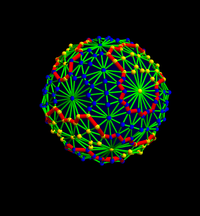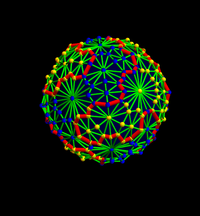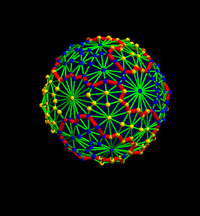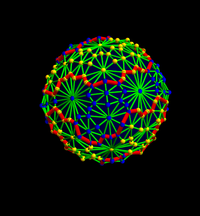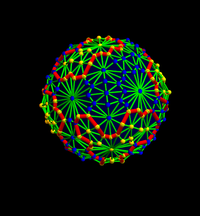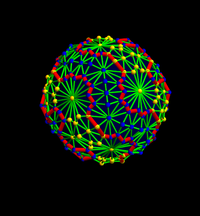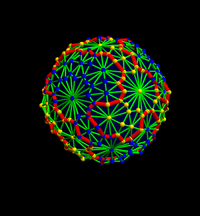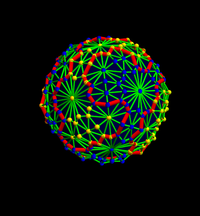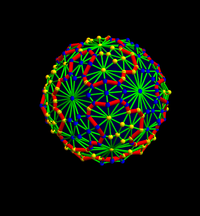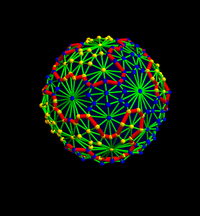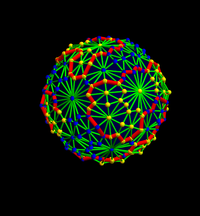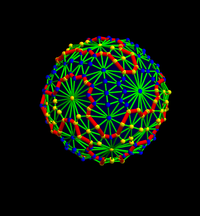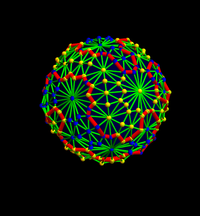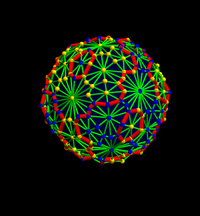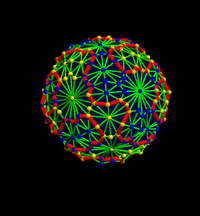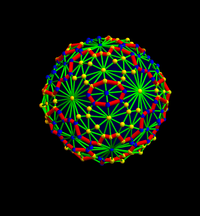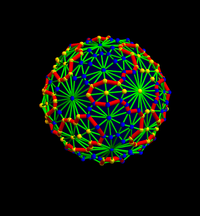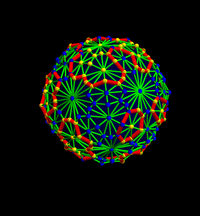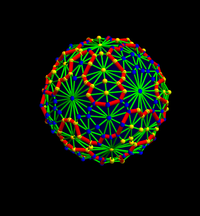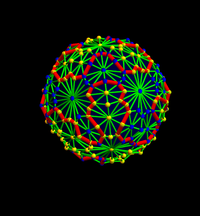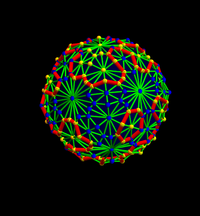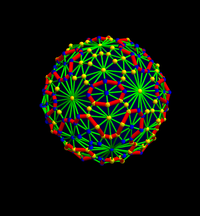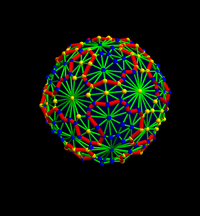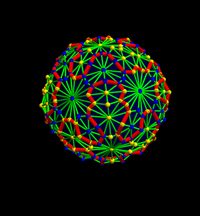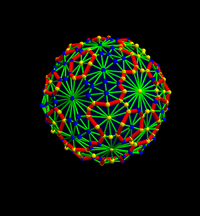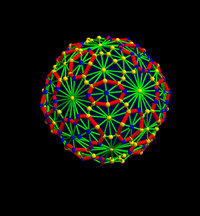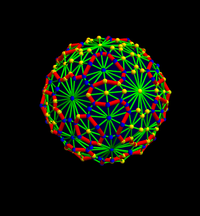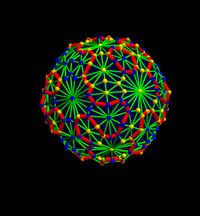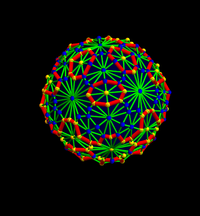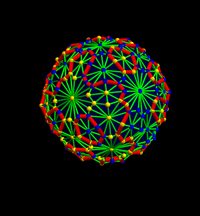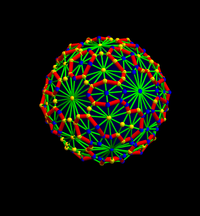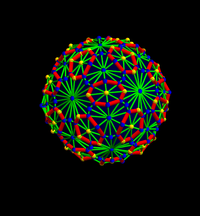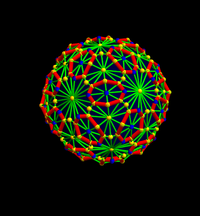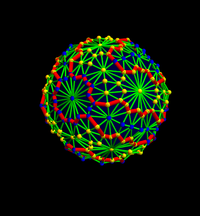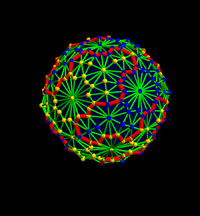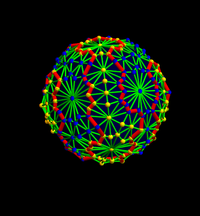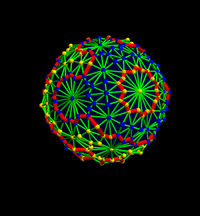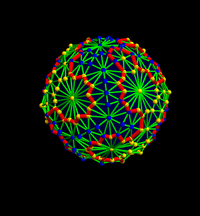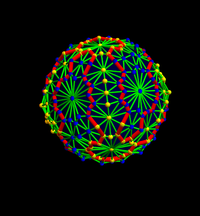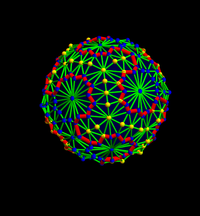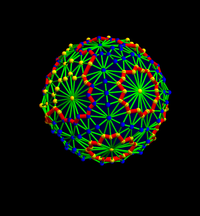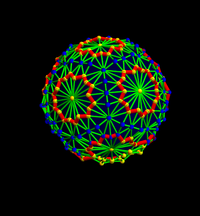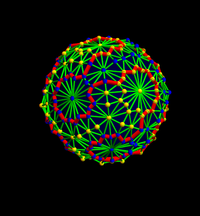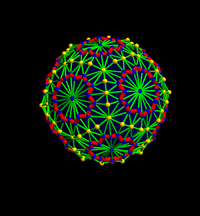# Some Chladni figures of an even more refined Icosahedron